Study Materials: ncert solutions

Our ncert solutions for Exercise 5.2 Class 8 maths 5. Data Handling - ncert solutions - Toppers Study is the best material for English Medium students cbse board and other state boards students.

Solutions ⇒ Class 8th ⇒ Mathematics ⇒ 5. Data Handling

# Exercise 5.2 Class 8 maths 5. Data Handling - ncert solutions - Toppers Study

Topper Study classes prepares ncert solutions on practical base problems and comes out with the best result that helps the students and teachers as well as tutors and so many ecademic coaching classes that they need in practical life. Our ncert solutions for Exercise 5.2 Class 8 maths 5. Data Handling - ncert solutions - Toppers Study is the best material for English Medium students cbse board and other state boards students.

## Exercise 5.2 Class 8 maths 5. Data Handling - ncert solutions - Toppers Study

CBSE board students who preparing for class 8 ncert solutions maths and Mathematics solved exercise chapter 5. Data Handling available and this helps in upcoming exams 2023-2024.

### You can Find Mathematics solution Class 8 Chapter 5. Data Handling

• All Chapter review quick revision notes for chapter 5. Data Handling Class 8
• NCERT Solutions And Textual questions Answers Class 8 Mathematics
• Extra NCERT Book questions Answers Class 8 Mathematics
• Importatnt key points with additional Assignment and questions bank solved.

NCERT Solutions do not only help you to cover your syllabus but also will give to textual support in exams 2023-2024 to complete exercise 5.2 maths class 8 chapter 5 in english medium. So revise and practice these all cbse study materials like class 8 maths chapter 5.2 in english ncert book. Also ensure to repractice all syllabus within time or before board exams for ncert class 8 maths ex 5.2 in english.

See all solutions for class 8 maths chapter 5 exercise 5 in english medium solved questions with answers.

### Exercise 5.2 class 8 Mathematics Chapter 5. Data Handling

• Exercise 5.2 Class 8 Maths 5. Data Handling - Ncert Solutions - Toppers Study
• Class 8 Ncert Solutions
• Solution Chapter 5. Data Handling Class 8
• Solutions Class 8
• Chapter 5. Data Handling Exercise 5.2 Class 8

## Exercise 5.2 Class 8 maths 5. Data Handling - ncert solutions - Toppers Study

Exercise 5.2

1. A survey was made to find the type of music that a certain group of young people liked in       a city. Adjoining pie chart shows the finding of this survey.
1. If 20 people liked classical music, how many young people were surveyed?
2. Which type of music is liked by the maximum number of people?
3. If a cassette company were to make 1000 CD’s, how many of each type would they make?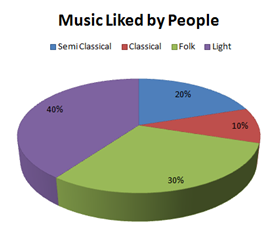•
1. Number of people who like classical music = 10 %

This 10% present 20 people.

So, 10% represent =  = 200 people.

Therefore, 200 young people were surveyed.

1. From the pie chart, it can be easily observed that the light music is represented by the maximum part of the pie chart 40%. Hence, most of the people like light music.
2.  Number of CD’s of classical music  = 10% of 1000

= 100

So, the number of CD’s of semi classical music = 20% of 100

=  = 200

So, the number of CD’s of folk music = 30%

=  = 300

And the number of cassettes of light music = 40% of  1000

=  = 400

2.   A group of 360 people were asked to vote for their favorite’s season from the three seasons rainy, winter and summer.

(i)   Which season got the most votes?

(ii)   Find the central angle of each sector.

(iii)   Draw a pie chart to show this information.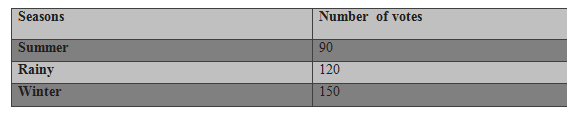1. Winter.
2. Total number of votes = 90 + 120+ 150 = 360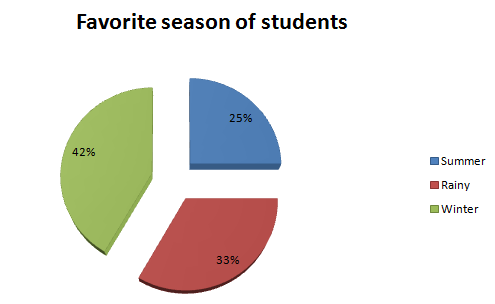Question 3:

Draw a pie chart showing the following information.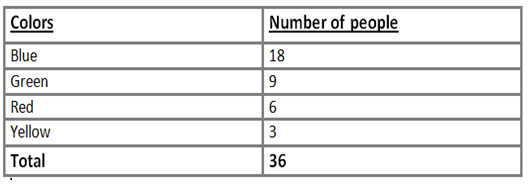Ans:

The central angle for each color can be calculated as follows.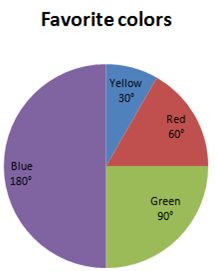Question:

The adjoining pie chart gives the mark scored in a examination by a student in Hindi, English, Mathematics, Social science and science. If the total marks obtained by a student were 540, answer the following question.

In which subject did the students score 105 marks?

How many more marks were obtained by the students in mathematics than Hindi?

Examine whether the sum of the marks obtained in social science and mathematics is more than that in science and Hindi.

(HINT: Just study the angle.)The central angle of each subject can be calculated as follows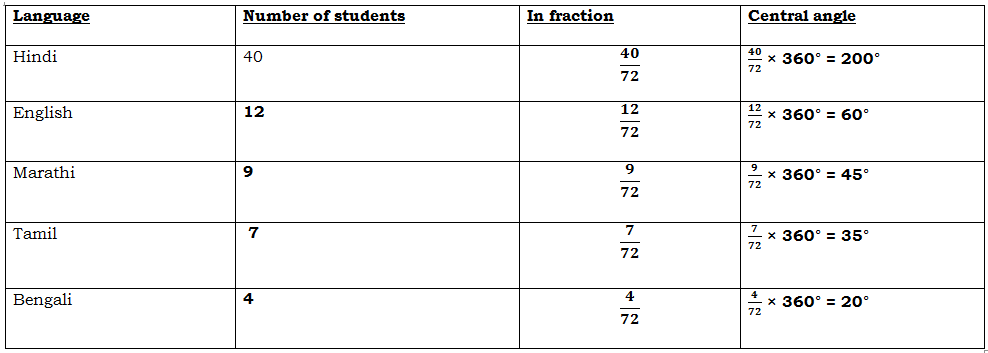A pie chart of the above data is as follows.##### Other Pages of this Chapter: 5. Data Handling

Important Study materials for classes 06, 07, 08,09,10, 11 and 12. Like CBSE Notes, Notes for Science, Notes for maths, Notes for Social Science, Notes for Accountancy, Notes for Economics, Notes for political Science, Noes for History, Notes For Bussiness Study, Physical Educations, Sample Papers, Test Papers, Mock Test Papers, Support Materials and Books.Mathematics Class - 11th

NCERT Maths book for CBSE Students.

books

## Study Materials List:

##### Solutions ⇒ Class 8th ⇒ Mathematics
1. Rational Numbers
2. Linear Equations in One Variable
4. Practical Geometry
5. Data Handling
6. Squares and Square Roots
7. Cubes and Cube Roots
8. Comparing Quantities
9. Algebraic Expressions and Identities
10. Visualising Solid Shapes
11. Mensuration
12. Exponents and Powers
13. Direct and Inverse Proportions
14. Factorisation
15. Introduction to Graphs
16. Playing with Numbers

New Books